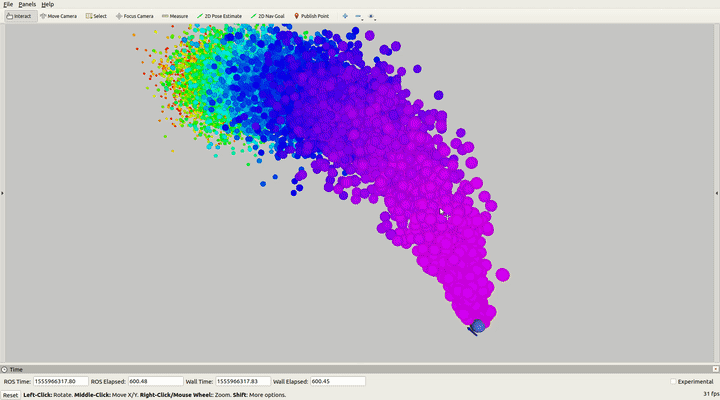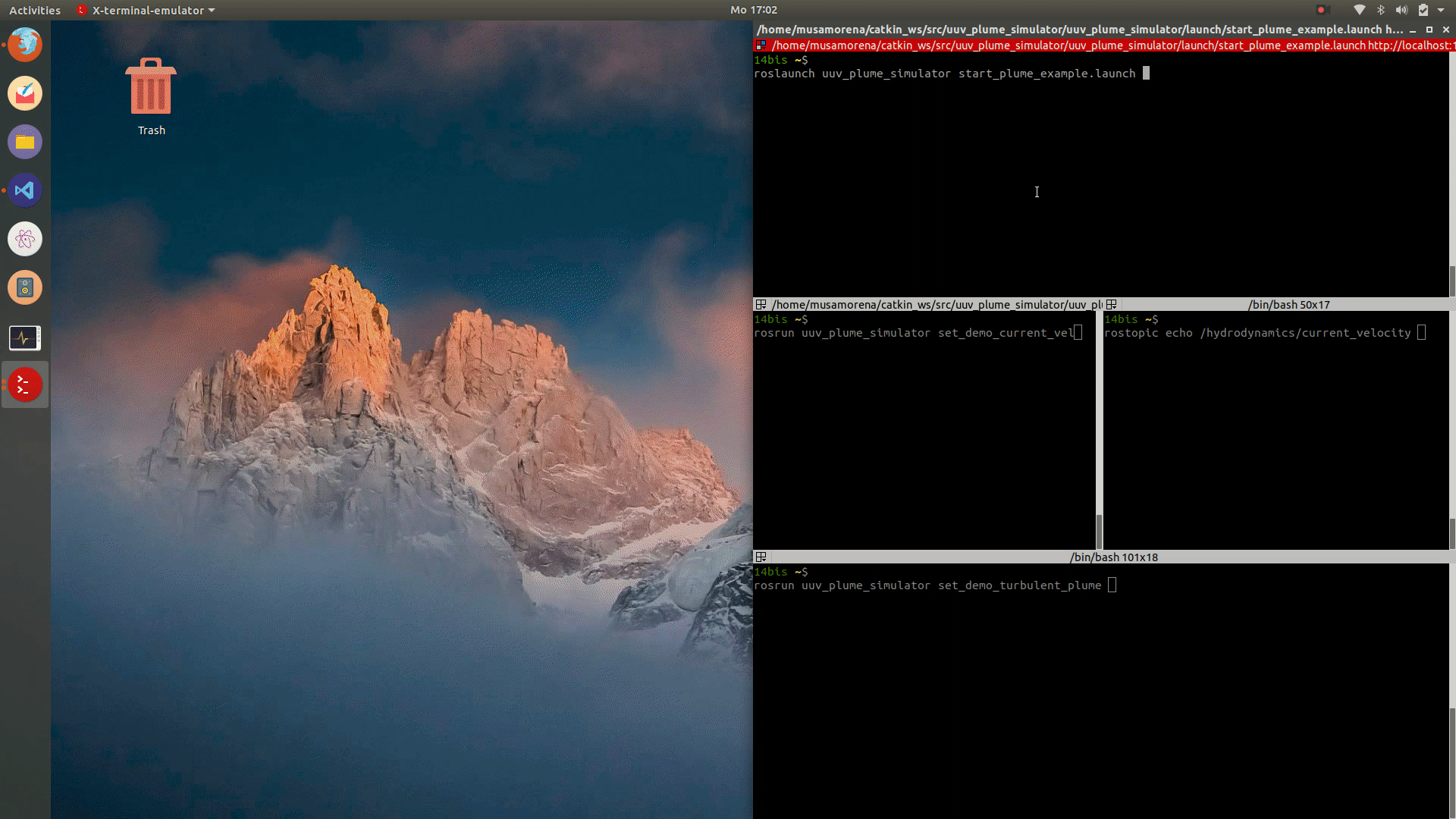# How to start a turbulent passive plumeTo start the simulation of a passive turbulent plume, first you need to start the plume server node

roslaunch uuv_plume_simulator start_plume_server.launch current_velocity_topic:=/hydrodynamics/current_velocity update_rate:=5


The current velocity topic must be generated either from a Gazebo underwater scenario running the underwater current ROS plugin (libuuv_underwater_current_ros_plugin.so). It must publish the current velocity as a geometry_msgs/Twist message. The current velocity will steer the plume and control its rise. Without any current, the plume particles will accumulate around the plume source.

#### Generation of the current velocity topic¶

##### Start the plume simulaton with Gazebo for current velocity generation¶

All Gazebo worlds included in the uuv_gazebo_worlds include the underwater current plugin and can be started as:

roslaunch uuv_gazebo_worlds ocean_waves.launch


A new world can be built at the same manner by including following snippet in the .world file

<plugin name="underwater_current_plugin" filename="libuuv_underwater_current_ros_plugin.so">
<namespace>hydrodynamics</namespace>
<constant_current>
<topic>current_velocity</topic>
<velocity>
<mean>0</mean>
<min>0</min>
<max>5</max>
<mu>0.0</mu>
<noiseAmp>0.0</noiseAmp>
</velocity>

<horizontal_angle>
<mean>0</mean>
<min>-3.141592653589793238</min>
<max>3.141592653589793238</max>
<mu>0.0</mu>
<noiseAmp>0.0</noiseAmp>
</horizontal_angle>

<vertical_angle>
<mean>0</mean>
<min>-3.141592653589793238</min>
<max>3.141592653589793238</max>
<mu>0.0</mu>
<noiseAmp>0.0</noiseAmp>
</vertical_angle>
</constant_current>
</plugin>


The current velocity will be published in /hydrodynamics/current_velocity and the same topic must be read by the plume server.

##### Start the current velocity server¶

Warning

Do not start the current velocity server node AND a Gazebo scenario. The topics will conflict with each other and be ovewritten.

To run the plume simulation independently from Gazebo, a current velocity server can be started by running

roslaunch uuv_plume_simulator start_current_velocity_server.launch current_velocity_topic:=/hydrodynamics/current_velocity


#### Start the passive turbulent plume¶

The can be started by using a plume server's service create_passive_scalar_turbulent_plume as follows:

rosservice call /create_passive_scalar_turbulent_plume "turbulent_diffusion_coefficients: {x: 0.05, y: 0.05, z: 0.05}
source: {x: -0.0, y: 0.0, z: -40.0}
buoyancy_flux: 0.05
stability_param: 0.001
n_points: 100000
max_particles_per_iter: 10
x_min: -200.0
x_max: 200.0
y_min: -75.0
y_max: 75.0
z_min: -50.0
z_max: 0.0
max_life_time: -1"


where

• turbulent_diffusion_coefficients are the coefficients used for the computation of the turbulent diffusion velocity components
• source is the position of the source of the plume in the ENU frame
• buoyancy_flux and stability_param are the parameters used for computation of the vertical buoyant velocity (see the previous tutorial for more details)
• n_points is the maximum number of particles allowed to exist at any time
• max_particles_per_iter is the maximum number of particles generated by the source at each iteration
• x_min, x_max, y_min, y_max, z_min, z_max are the limits of the bounding box where the particles are allowed to exist in
• max_life_time is the maximum amount of seconds each particle is allowed to exist before being destroyed (-1 for not limit, the particles will then only be destroyed when they reach the limits of the bounding box)

Note

The particles will be destroyed when reaching the bounding box's walls, except if the upper box limit is equal or above $z=0$, in which case the position of the particle will be always the minimum between 0 and the current $z$ position wrt the ENU frame.

#### Running the example¶

The full tutorial to start a turbulent plume can be started with the following commands:

roslaunch uuv_plume_simulator start_plume_example.launch

rosrun uuv_plume_simulator set_demo_current_vel

rosrun uuv_plume_simulator set_demo_turbulent_plume